Скачать презентацию Math Activity 1 Answer the

39e85cf45ca65cceb6be7daa20152177.ppt

• Количество слайдов: 8Math Activity 1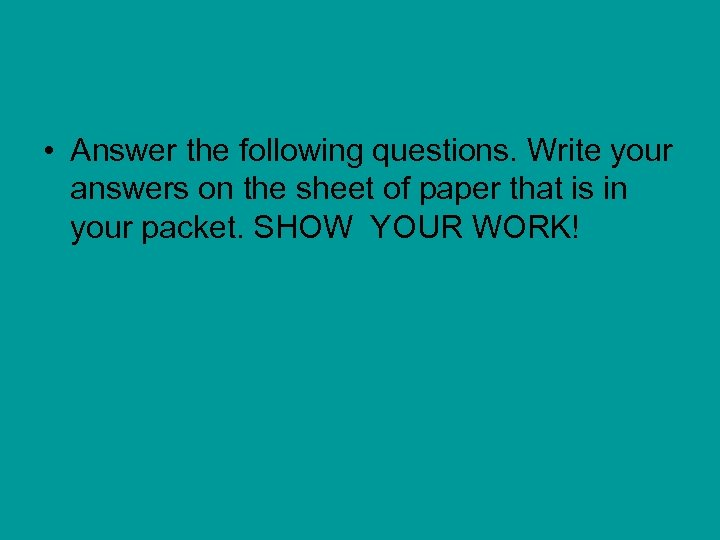• Answer the following questions. Write your answers on the sheet of paper that is in your packet. SHOW YOUR WORK!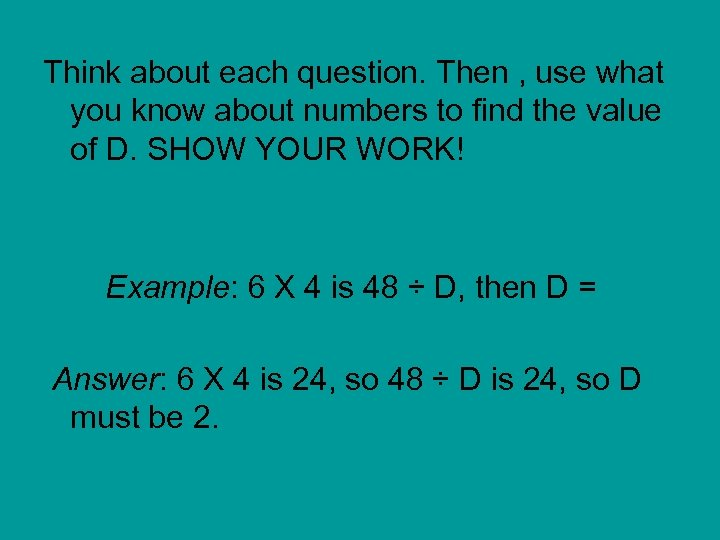Think about each question. Then , use what you know about numbers to find the value of D. SHOW YOUR WORK! Example: 6 X 4 is 48 ÷ D, then D = Answer: 6 X 4 is 24, so 48 ÷ D is 24, so D must be 2.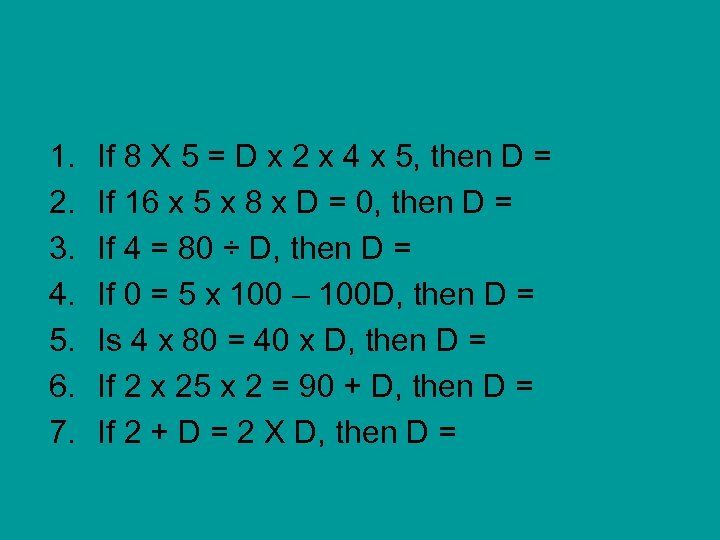1. 2. 3. 4. 5. 6. 7. If 8 X 5 = D x 2 x 4 x 5, then D = If 16 x 5 x 8 x D = 0, then D = If 4 = 80 ÷ D, then D = If 0 = 5 x 100 – 100 D, then D = Is 4 x 80 = 40 x D, then D = If 2 x 25 x 2 = 90 + D, then D = If 2 + D = 2 X D, then D =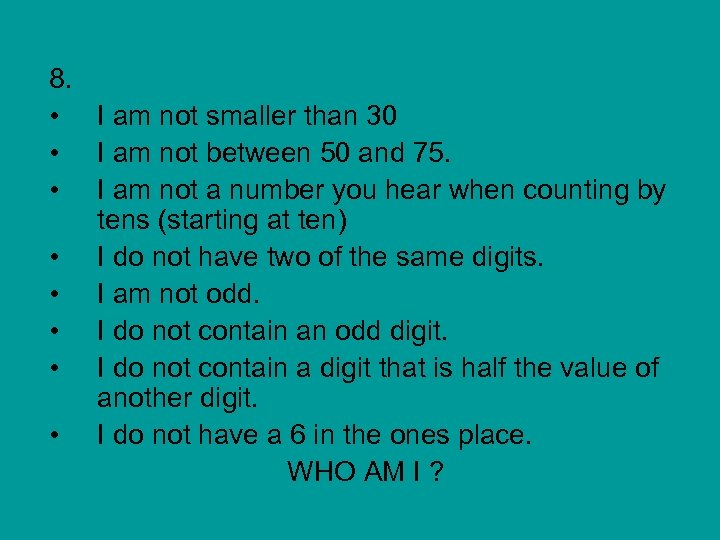8. • I am not smaller than 30 • I am not between 50 and 75. • I am not a number you hear when counting by tens (starting at ten) • I do not have two of the same digits. • I am not odd. • I do not contain an odd digit. • I do not contain a digit that is half the value of another digit. • I do not have a 6 in the ones place. WHO AM I ?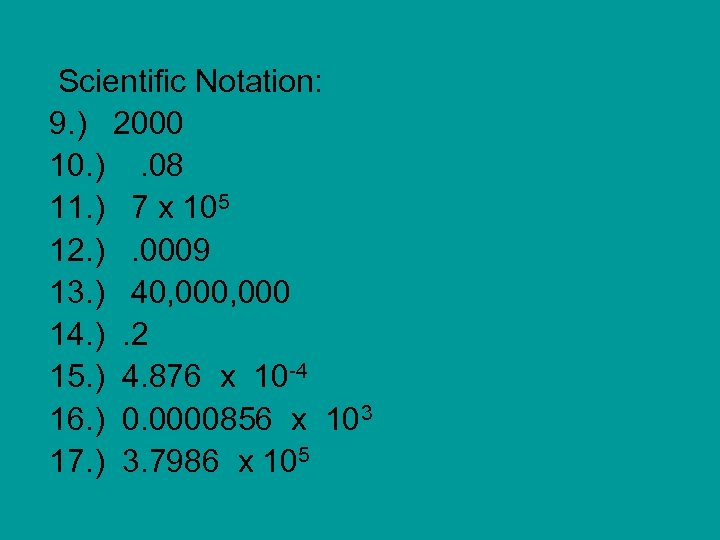Scientific Notation: 9. ) 2000 10. ). 08 11. ) 7 x 105 12. ). 0009 13. ) 40, 000 14. ). 2 15. ) 4. 876 x 10 -4 16. ) 0. 0000856 x 103 17. ) 3. 7986 x 105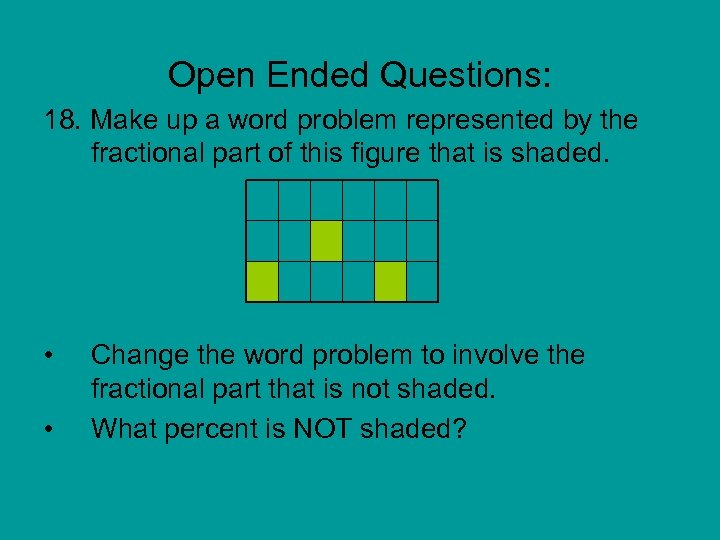Open Ended Questions: 18. Make up a word problem represented by the fractional part of this figure that is shaded. • • Change the word problem to involve the fractional part that is not shaded. What percent is NOT shaded?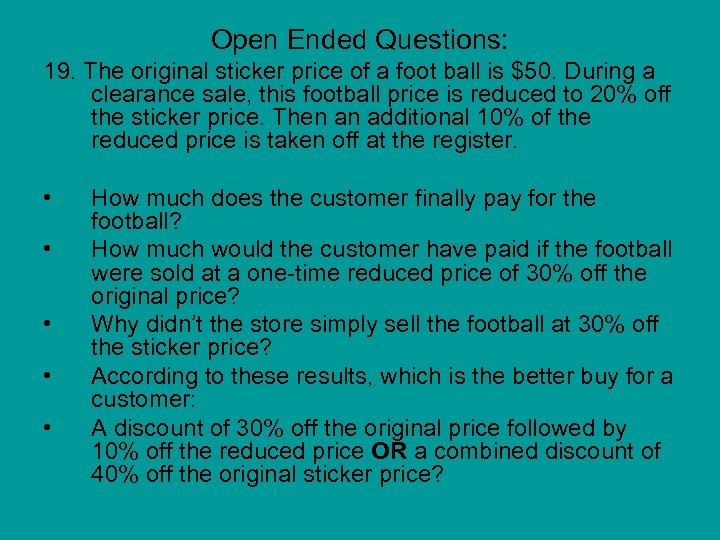Open Ended Questions: 19. The original sticker price of a foot ball is \$50. During a clearance sale, this football price is reduced to 20% off the sticker price. Then an additional 10% of the reduced price is taken off at the register. • • • How much does the customer finally pay for the football? How much would the customer have paid if the football were sold at a one-time reduced price of 30% off the original price? Why didn’t the store simply sell the football at 30% off the sticker price? According to these results, which is the better buy for a customer: A discount of 30% off the original price followed by 10% off the reduced price OR a combined discount of 40% off the original sticker price?